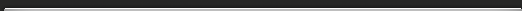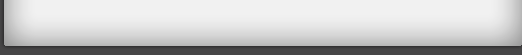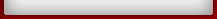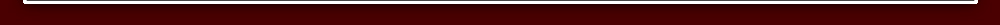HomeWelcome to VEDIC MAGIC---Maths guru Here you can learn to calculate as fast as ur calculator....That too with out use of Pen & Paper... So are you ready??? Browse through the different tutorials..Listed on navigation menu..& Practice the tricks. Remember PRACTICE MAKES MAN PERFECT... & ALSO WOMEN Below is the list of What you will be learning in Each Tutorial..Click on Tutorial to study.. Tutorial 1>ALL FROM 9 AND THE LAST FROM 10 to perform instant subtractions. Tutorial 2>VERTICALLY AND CROSSWISE for multiplying numbers. Tutorial 3>The easy way to add and subtract fractions. Tutorial 4>A quick way to square numbers that end in 5. Tutorial 5>An elegant way of multiplying. Tutorial 6>Multiplying a number by 11. Tutorial 7>Method for dividing by 9. Tutorial 8>Vedic Maths - Tips & Tricks. marketing But before starting..... One Question Can you prove 3=2 ?? I hope no. But Ramanujam had.. How?? Here is how... See this illustration: -6 = -6 9-15 = 4-10 Adding 25/4 to both sides: 9-15+(25/4) = 4-10+(25/4 ) Changing the order 9+(25/4)-15 = 4+(25/4)-10 [This is just like : a2+b2-2ab=(a-b)2.] Here a = 3, b=5/2 for L.H.S....... and a =2, b=5/2 for R.H.S. So it can be expressed as follows (3-5/2)(3-5/2) = (2-5/2)(2-5/2) Taking positive square root on both sides. 3 - 5/2 = 2 - 5/2 3 = 2 Can u find any flaws?? how was that??cool isn't it!! marketing